# DATABASE JOINS

Database Joins | DBMS Tutorial | Minigranth

# Database Joins : Introduction

• Database joins are the alternate to the Cartesian product operation of the relational algebra concept.
• But, the logic behind the data set joins is same as that of a Cartesian product but, joins in database includes the option for condition which needs to be satisfied in order to get the desired results/output.
• The database joins has the ability of combining two or more data tables/tuples into a single table/table only if the following conditions are satisfied.
• There must be a common attribute in both(tables which are participating) tables.
• Join condition must be satisfied.
• Database joins can be broadly classified into two categories which are further categorized into sub categories. All of them are explained below.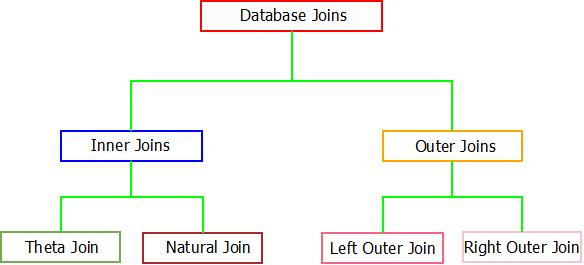Database Joins : Classification

### Database Joins : The Inner Joins

• The idea behind inner join is very simple. When inner join is applied to tuples or tables, only those tuples of the table are kept which have common attribute in all the tables. Other tuples which are not common are dropped from the resulting tuple/table.
• To implement this concept two possible inner joins are available i.e. Theta Join & Natural Join.

1. Theta Join

• If a condition is satisfied by the participating tables from different relations, then the tuples are combined together using Theta Join. Theta join is denoted through “Theta(Θ)”.
 Syntax : R1(X1, X2,X3…Xn)  ⋈(Condition “θ”) R2(Y1, Y2,Y3…Yn) where, R1 and R2 are relations having (X1, X2,X3…Xn)  and (Y1, Y2,Y3…Yn) as attributes respectively.

For example : Consider the tables Student_Details and Student_Result. Now, if we want to implement theta join on these relations, the result will look like:Database joins : Theta Join

2. Natural Join

• Natural join does not supports any condition such as theta join and works only if, one attribute or more than one attributes are common between the joining/participating relations.
 Syntax : R1(X1, X2,X3…Xn)  ⋈ R2(Y1, Y2,Y3…Yn) where, R1 and R2 are relations having (X1, X2,X3…Xn)  and (Y1, Y2,Y3…Yn) as attributes respectively.

For example : Consider the tables Student_Details and Student_Result. Now, if we want to implement natural join on these relations, the result will look like:Database joins : Natural Join

### Database Joins : The Outer Joins

• Outer join overcomes the inability of inner joins of dropping the tuples which are uncommon among participating relations. If we want to display those tuples which are not common, the concept of outer join is used.
• Also, if all the tuples needs to be displayed from all the participating relations, outer joins can be used. They are of three types : Left Outer Join, Right Outer Join & Full Outer Join.

1. Left Outer Join

• There exists a concept of position(left or right) of relations in case of both left and right outer join.
• To implement left outer join, at least one entity needs to be common in the relations. All the attributes/tuples present in the left relation are recorded in the resulting relation along with those which are commonly present in the right relation.
• If in case any tuple in left relation does not matches with the tuple in right relation, NULL value will be displayed against that tuple in the resulting relation.
 Syntax : R1(X1, X2,X3…Xn)R2(Y1, Y2,Y3…Yn) where, R1(Left Relation) and R2(Right Relation) are relations having (X1, X2,X3…Xn)  and (Y1, Y2,Y3…Yn) as attributes respectively and "” denotes left outer join.

For example :  Consider the tables Student_Details and Student_Result. Now, if we want to implement left outer join on these relations, the result will look like: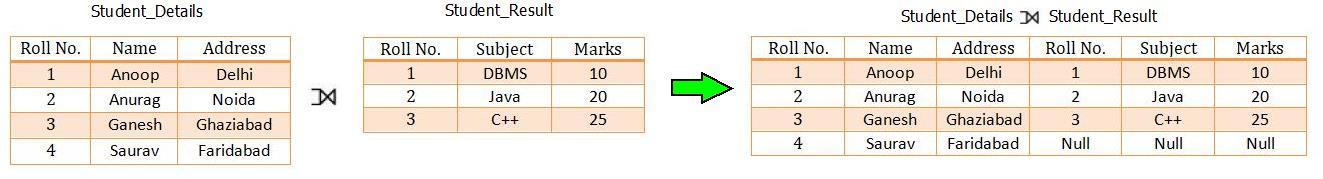DBMS Joins : Left Outer Join

2. Right Outer Join

• There exists a concept of position(left or right) of relations in case of both left and right outer join.
• The right outer join is completely similar to left outer join except the resulting relation will include all the tuples from relation present on right hand relation.
• Also, NULL value will be displayed against the tuple which doesn’t matches up with the left side relation.
 Syntax : R1(X1, X2,X3…Xn)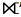R2(Y1, Y2,Y3…Yn) where, R1(Left Relation) and R2(Right Relation) are relations having (X1, X2,X3…Xn)  and (Y1, Y2,Y3…Yn) as attributes respectively and "” denotes right outer join.

For example : Consider the tables Student_Details and Student_Result. Now, if we want to implement right outer join on these relations, the result will look like: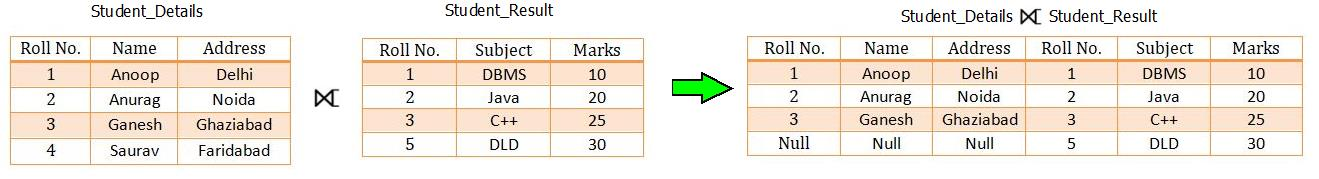Database Joins : Right Outer Join

3. Full Outer Join

• In full outer join, both the relations are merged together which results in a relation consisting of all the tuples.
• If in case, tuples doesn’t matches, NULL value is passes against that.
 Syntax : R1(X1, X2,X3…Xn)R2(Y1, Y2,Y3…Yn) where, R1 and R2 are relations having (X1, X2,X3…Xn)  and (Y1, Y2,Y3…Yn) as attributes respectively and “” denotes full outer join.

For example : Consider the tables Student_Details and Student_Result. Now, if we want to implement full outer join on these relations, the result will look like: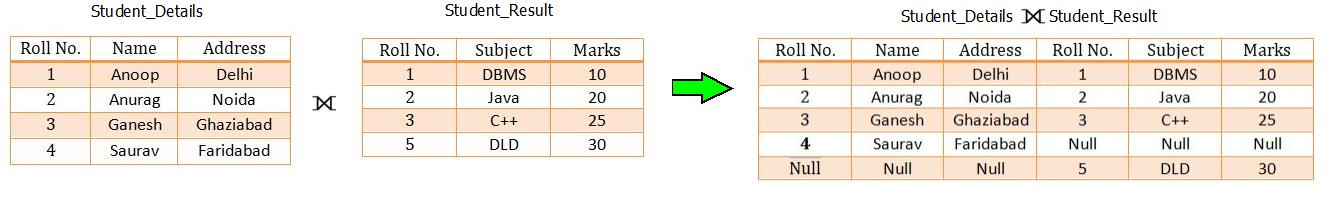Database Joins : Full Outer Join